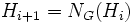# Finitarily hypernormalized subgroup

BEWARE! This term is nonstandard and is being used locally within the wiki. [SHOW MORE]
This article defines a subgroup property: a property that can be evaluated to true/false given a group and a subgroup thereof, invariant under subgroup equivalence. View a complete list of subgroup properties[SHOW MORE]

## Definition

A subgroup$H$ of a group$G$ is termed a finitarily hypernormalized subgroup if the iterated sequence of normalizers, starting from$H$, reaches$G$ in finitely many steps. In other words, define a sequence$H_i$ by:

•$H_0 = H$.
•$H_{i+1} = N_G(H_i)$.

Then,$H$ is$k$-hypernormalized if$H_k = G$ for some natural number$k$. If there exists a natural number$k$ such that$H$ is$k$-hypernormalized, we say that$H$ is finitarily hypernormalized.

## Relation with other properties

### Weaker properties

• Subnormal subgroup: In fact, a$k$-hypernormalized subgroup is$k$-subnormal. However, a$k$-subnormal subgroup need not be finitarily hypernormalized, and even if it is, it need not be$k$-normalized. For full proof, refer: Abnormal normalizer and 2-subnormal not implies normal, normalizer of 2-subnormal subgroup may have arbitrarily large subnormal depth
• Hypernormalized subgroup

### Related group properties

• Hypernormalizing group is a group in which every ascendant subgroup is hypernormalized. A finite hypernormalizing group, or more generally, a slender hypernormalizing group, must have every subnormal subgroup is finitarily hypernormalized.
• In a nilpotent group every subgroup is hypernormalized, but a$k$-subnormal subgroup need not be$k$-hypernormalized.

## Metaproperties

### Transitivity

NO: This subgroup property is not transitive: a subgroup with this property in a subgroup with this property, need not have the property in the whole group
ABOUT THIS PROPERTY: View variations of this property that are transitive|View variations of this property that are not transitive
ABOUT TRANSITIVITY: View a complete list of subgroup properties that are not transitive|View facts related to transitivity of subgroup properties | View a survey article on disproving transitivity

### Intermediate subgroup condition

NO: This subgroup property does not satisfy the intermediate subgroup condition: it is possible to have a subgroup satisfying the property in the whole group but not satisfying the property in some intermediate subgroup.
ABOUT THIS PROPERTY: View variations of this property satisfying intermediate subgroup condition | View variations of this property not satisfying intermediate subgroup condition
ABOUT INTERMEDIATE SUBGROUP CONDITION: View other subgroup properties not satisfying intermediate subgroup condition| View facts about intermediate subgroup condition